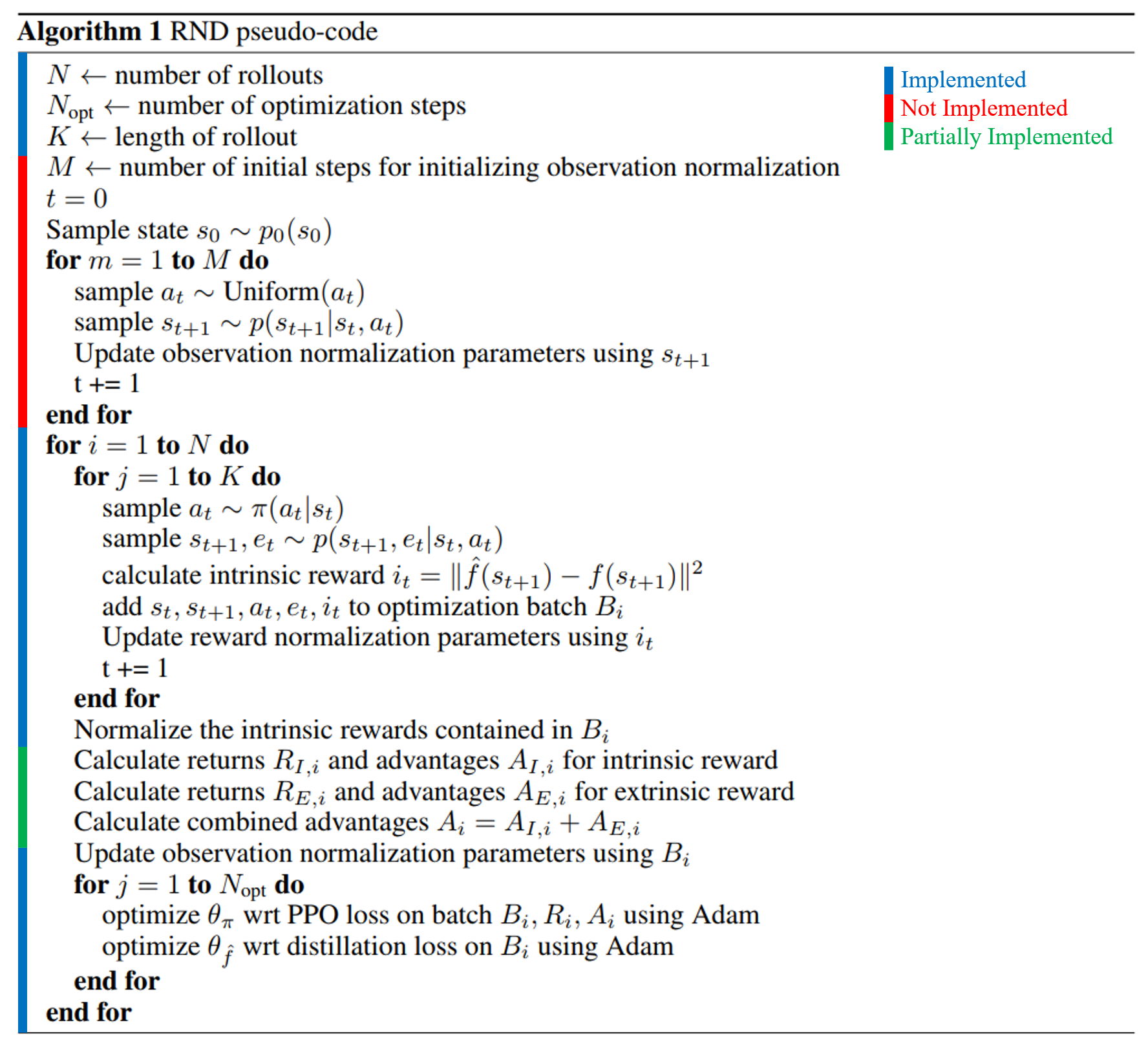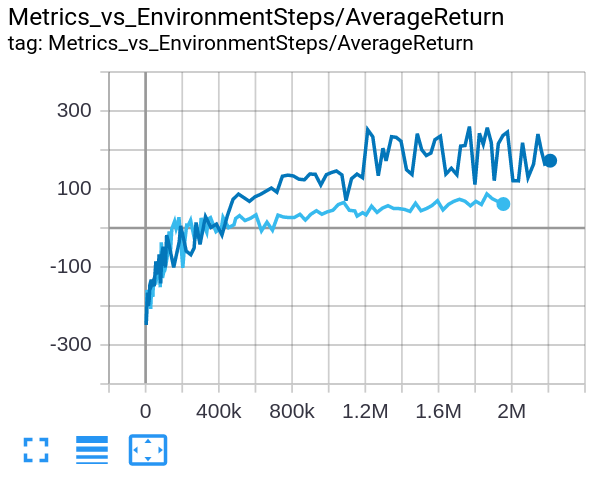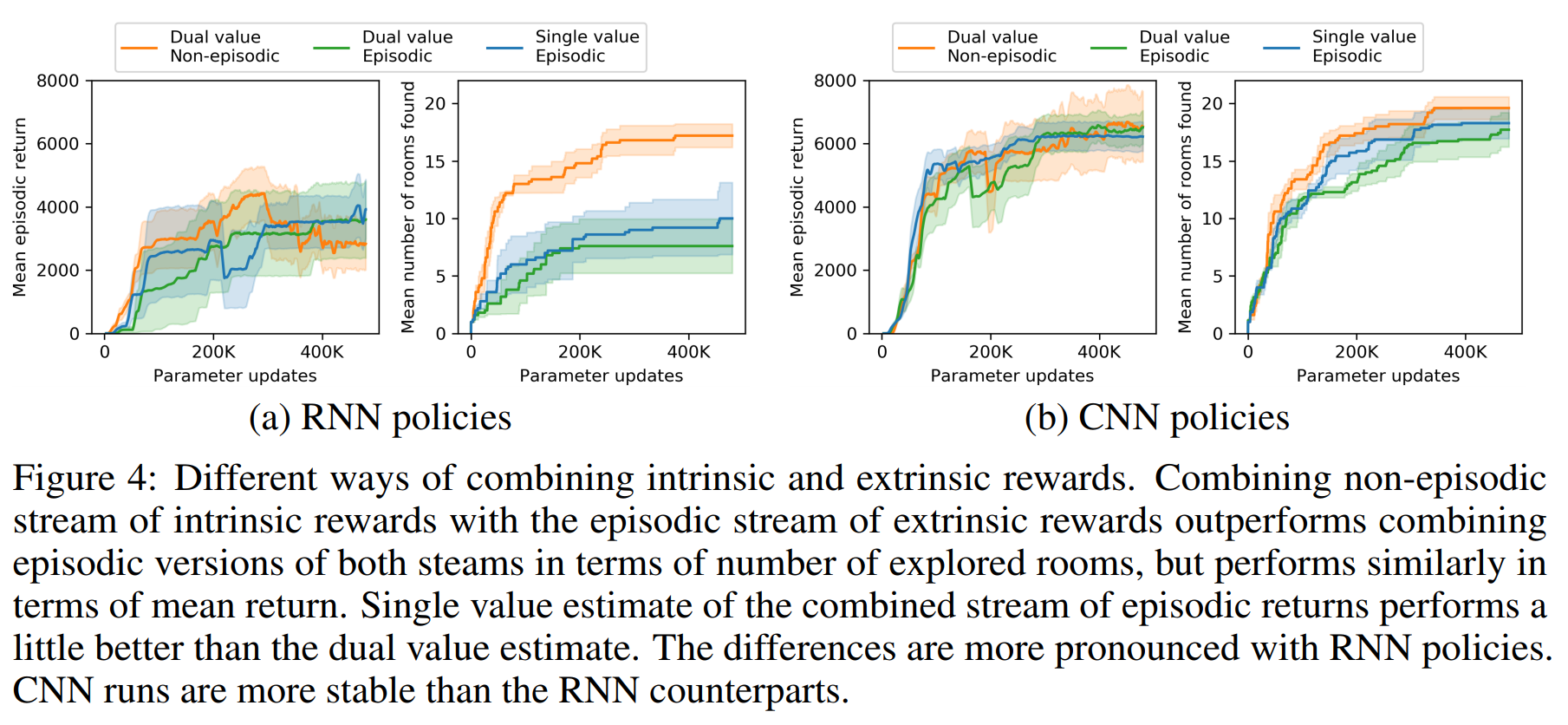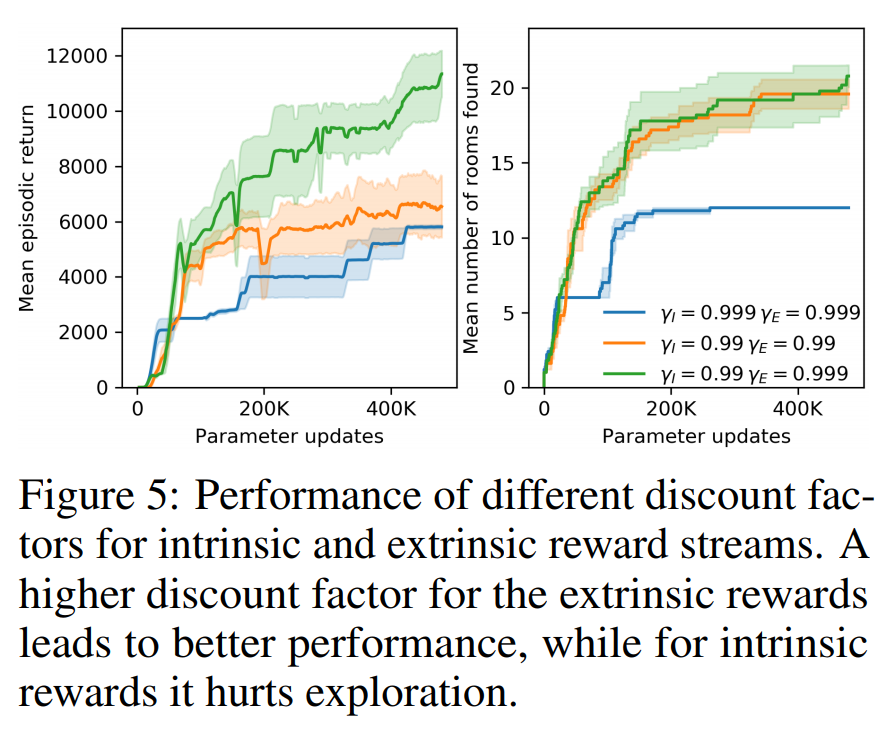Since my last post, I have been implementing and testing Random Network Distillation (RND) by Burda et al. As I was able to implement the most important parts of RND, in this post I give a brief summary of how I implemented RND.

## Implemented

First, here is an image that approximately summarizes what has been implemented:The main parts are computing the intrinsic reward (RND loss) and updating the RND networks. Because the full code is too long to put in this post, I selected the segments that are most relevant to the implementation.

### Defining RND Materials

The intrinsic reward for RND is defined as a MSE loss of two vectors: predictor and target. Both vectors are outputs of two networks with the same architecture - the RND predictor network and the RND target network.

The RND predictor network and RND target network is defined in train_eval.py where other networks are also defined.

if use_rnd:
rnd_net = encoding_network.EncodingNetwork(
tf_env.observation_spec(),
fc_layer_params=value_fc_layers,
name='PredictorRNDNetwork')
target_rnd_net = encoding_network.EncodingNetwork(
tf_env.observation_spec(),
fc_layer_params=rnd_layers,
name='TargetRNDNetwork')


The optimizer and loss function for RND is also defined in the next few lines. RND uses Adam optimizer and Mean Squared Loss (MSE) function.

if use_rnd:
learning_rate=learning_rate
)
rnd_loss_fn = ppo_agent.mean_squared_loss


### Computing Intrinsic Reward

Then, the PPOAgent has a new function rnd_loss() that returns the RND loss given the normalized observations. First, The RND prediction and target is calculated by inputting observations to the relevant RND network. Then, the RND loss is calculated using the saved rnd_loss_fn.

# Prediction and Target have shape (# Env, # Timesteps, Final FC Layer)
rnd_prediction, _ = self._rnd_network(time_steps.observation)
rnd_target, _ = self._target_rnd_network(time_steps.observation)
# rnd_losses have shape (# Env, # Timesteps)
rnd_losses = self._rnd_loss_fn(rnd_prediction, rnd_target, axis=2)
avg_rnd_loss = tf.reduce_mean(rnd_losses)


Before using them as intrinsic rewards, the agent needs to normalize them. This is done through a _rnd_reward_normalizer:

self._rnd_reward_normalizer = None
if rnd_normalize_rewards:
self._rnd_reward_normalizer = tensor_normalizer.StreamingTensorNormalizer(
tensor_spec.TensorSpec([], tf.float32), scope='normalize_rnd_reward')


Then, the agent uses these normalized loss as intrinsic rewards to compute returns and advantages.

intrinsic_rewards, _ = self.rnd_loss(normalized_observations, debug_summaries=self._debug_summaries)
next_time_steps, value_preds, intrinsic_rewards=intrinsic_rewards)


Of course, the reward normalizer is updated after each update epoch.

# Update RND reward normalizer
if self._use_rnd and self._rnd_reward_normalizer:
# Use normalized observations when computing RND loss
normalized_observations = self._observation_normalizer.normalize(time_steps.observation, clip_value=5)
intrinsic_rewards, _ = self.rnd_loss(normalized_observations, debug_summaries=self._debug_summaries)
self._rnd_reward_normalizer.update(intrinsic_rewards, outer_dims=[0, 1])


### Training RND Target Network

The purpose of intrinsic reward is to numerically represent the novelty of the input observation. Thus, by training the RND predictor network to minimize the MSE loss between the output of RND target network and RND predictor network, the RND predictor network minimizes intrinsic rewards (MSE loss) for observation (inputs) that are frequently seen.

The training code is very simple thanks to TensorFlow, and the training is done after the policy network is trained.

rnd_variables_to_train = self._rnd_network.trainable_weights
# Tuple is used for py3, where zip is a generator producing values once.


self._rnd_optimizer.apply_gradients(


### Verifying RND in LunarLander-v2

To check if RND is working correctly before testing it on benchmark environment, I ran RND on LunarLander-v2 and compared it with baseline: PPO.The dark blue line denotes RND, and the light blue line denotes PPO. In this comparison, PPO was stuck in a local minima around zero, whereas RND was able to escape this local minima and receive a higher score.

Of course, a single-seed comparison cannot be used to argue the correctedness of my RND implementation. However, it gave me a peace of mind!

## To Be Implemented

Although the basic idea of RND is simple, there are a lot of “tricks” that the authors mention that can further improve the performance of the agent. Some of them have been implemented already, but here are some that have not yet been implemented.

### Recurrence

Section 3.5 discusses using an RNN policy to mitigate the problems of partially observable environments. However, the authors show in Figure 4 that using RNN did not improve performance, so I plan on implementing this later, although implementing recurrence for RND should be simple since TF-Agents already supports recurrence for PPO.Figure 4 from Exploration by Random Network Distillation (Burda et al., 2018)

Section 2.3 discusses Combining Intrinsic and Extrinsic Returns. They use separate value heads $V_E$ and $V_I$, which allows for different training settings for extrinsic and intrinsic rewards. Notably, when calculating $V_I$, the authors suggest reconsidering the environment as a non-episodic one. The authors also report better results when using higher discount factor of $\gamma_E = 0.999$ when computing $V_E$.

Currently, a single value head is used for both rewards. Figure 4 (above) shows that making intrinsic reward setting non-episodic does not raise performance, so this will be implemented later. However, Figure 5 (below) shows that using a different discount factor does raise performance, so I will implement this first.Figure 5 from Exploration by Random Network Distillation (Burda et al., 2018)

### Observation Clipping

Section 2.4 discusses Reward and Observation Normalization. The paper specifies that intrinsic rewards were normalized by “dividing it by a running estimate of the standard deviations of the intrinsic returns,” and the same was done for observations used as inputs to the RND target and predictor networks. The normalization parameters are initialized using a random agent before training. The normalized observation is also clipped to be between [-5, 5].

Currently, extrinsic rewards, intrinsic rewards, and observations are all normalized through tensor_normalizer.StreamingTensorNormalizer. The observation is not clipped.

## Modifying Hyperparameters

Like any other fields of deep learning, hyperparameter tuning is crucial for performance in deep reinforcement learning. To reproduce the results in the paper, I decided to list all the hyperparameters specified in the paper and compare it with the default hyperparameters in TF-Agents.

Hyperparameters Paper TF-Agents
Preprocessing
Grey-scaling True
Observation downsampling (84, 84)
Extrinsic reward clipping
reward_norm_clipping
[-1, 1] [-10, 10]
Intrinsic reward clipping False False
Max frames per episode 18K
Terminal on loss of life False
Skip frames ? 2~4
Random starts False False
Sticky action probability 0.25 0.25
Training
Number of parallel environment
num_parallel_environments
128 30
Proportion of experience used for training predictor 0.25 1
Rollout length 128
Total number of rollouts per env 30K
Number of minibatches 4 1
Number of optimization epochs
num_epochs
4 25
Coefficient for extrinsic reward 2 1
Coefficient for intrinsic reward 1 1
Learning rate
learning_rate
0.0001 0.0001
Optimization algorithm
optimizer, rnd_optimizer
GAE
use_gae
Yes No
$\lambda$
lambda_value
0.95 N/A
Entropy coefficient
entropy_regularization
0.001 0
$\gamma_E$
discount_factor
0.999 0.99
$\gamma_I$ 0.99 0.99
PPO Style Clip Penalty
Clip range
importance_ratio_clipping
[0.9, 1.1] -
initial_adaptive_kl_beta